# GRAPHSP – Customized algorithms for topology identification and optimal design of noisy networks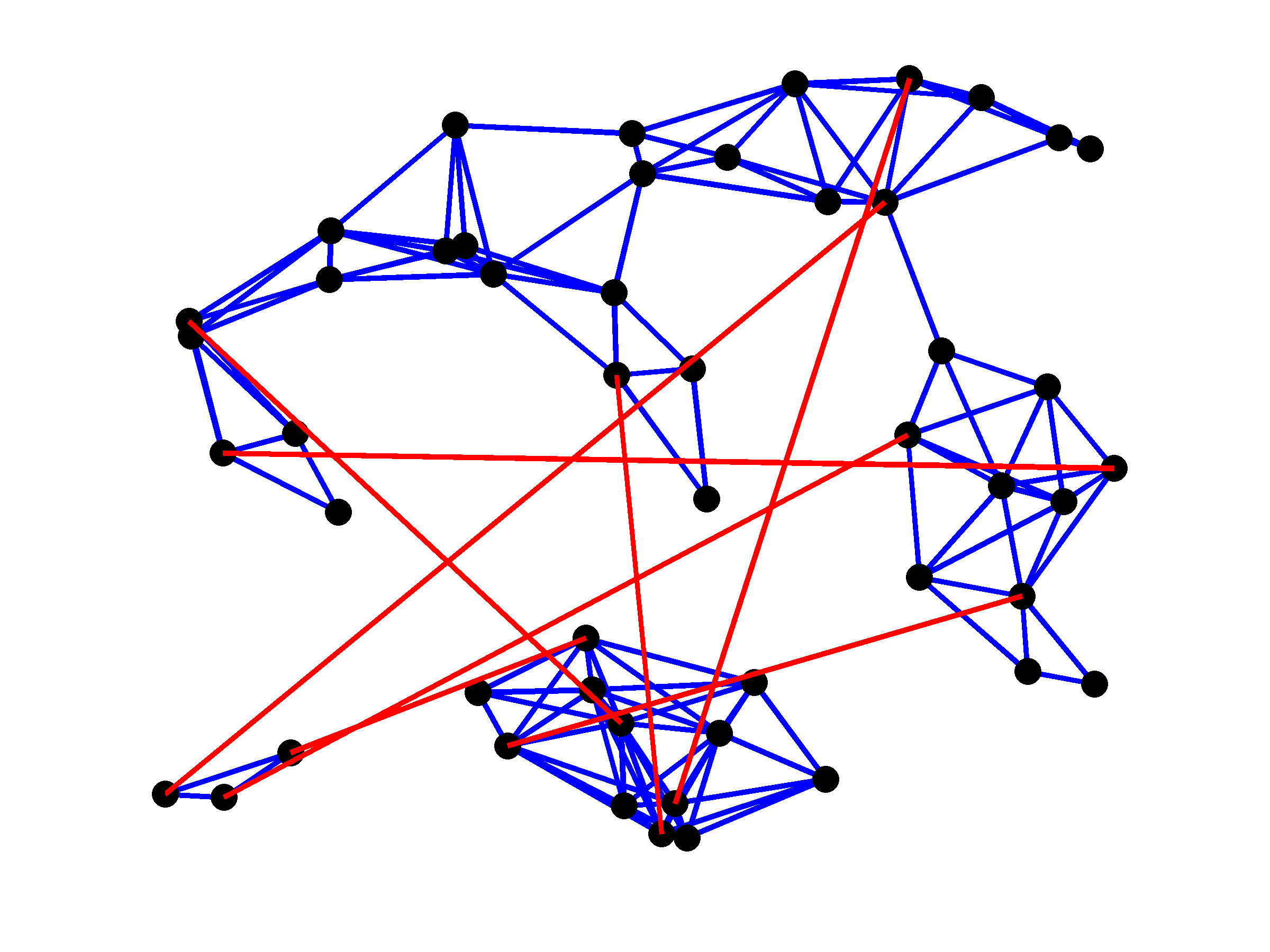May 2016 Matlab Files Download all Matlab files Paper arXiv:1506.03437v2

## Purpose

This website provides a Matlab implementation of customized algorithms for topology identification and optimal design of undirected consensus networks with additive stochastic disturbances. By introducing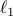-regularization into the optimal control formulation aimed at minimizing the steady-state variance amplification, this problem can be cast as a semidefinite program. Standard interior-point (IP) method solvers can be used to compute the optimal solution for small- and medium-size networks. We derive a Lagrange dual and exploit structure of the optimality conditions for undirected networks to develop three customized algorithms that are well-suited for large problems. Our customized algorithms are based on:

• the infeasible primal-dual IP method;

• the proximal Newton method.

The proximal gradient algorithm is a simple first-order method that updates the controller graph Laplacian via convenient use of the soft-thresholding operator. In the IP method, the Newton direction is obtained using an inexact iterative procedure based on the preconditioned conjugate gradients and, in the proximal Newton method it is computed using cyclic coordinate descent over the set of active variables. We illustrate that all of our algorithms significantly outperform the general-purpose solvers and greedy strategies and that the proximal algorithms can solve the problems with more than million edges in the controller graph in a few minutes, on a PC. We also exploit structure of connected resistive networks to demonstrate how additional edges can be systematically added in order to minimize the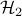norm of the closed-loop system.

## Problem formulation

We consider a control problem for undirected consensus networks with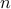nodes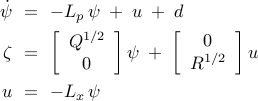where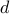and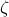denote disturbance input and performance output,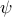is the state of the network, and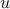is the control input. Symmetric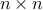matrices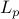and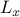are Laplacians of the plant and the controller, and matrices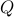and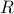denote state and control weights in the optimal control problem. Upon closing the loop we obtain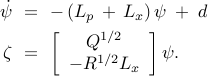Our objective is to identify the optimal topology forand to design the corresponding edge weights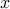in order to achieve the desired tradeoff between controller sparsity and network performance. The performance is quantified by the steady-state variance amplification of the stochastically-forced network (from the white-in-time inputto the performance outputwhich penalizes deviation from consensus and control effort).

To achieve consensus in the absence of disturbances, the closed-loop network has to be connected. This amounts to positive definiteness of the ‘‘strengthened’’ graph Laplacian of the closed-loop network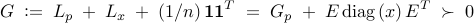where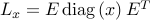,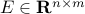is the given incidence matrix of the controller graph,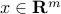is the vector of controller edge weights, and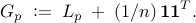Structural restrictions on the Laplacian matrices introduce an additional constraint on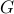,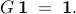## Sparsity-promoting optimal control problem

The steady-state amplification of white-in-time disturbances is determined by thenorm of stochastically-forced network,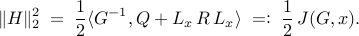The objective function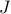can be expressed as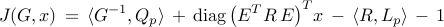where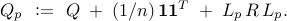The problem of designing a controller graph that provides an optimal tradeoff between theperformance of the closed-loop network and the controller sparsity can be formulated as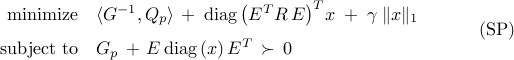where thenorm of,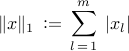is introduced as a proxy for inducing sparsity. In (SP), the positive definite matrix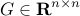and the vector of the edge weights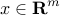are optimization variables; the problem data is given by the positive regularization parameter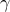, the plant graph Laplacian, the state and control weightsand, and the incidence matrix of the controller graph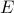.

Since minimization of the Lagrangian associated with (SP) does not lead to an explicit expression for the dual function, we introduce an auxiliary variableand find the dual of. It is well-known that the consensus can be achieved even if some edge weights take negative values; e.g., see Xiao and Boyd ’04. By expressing the vector of the edge weights,, as a difference between two non-negative vectors,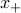and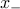,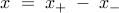the optimal control problem can be expressed asThe Lagrange dual of the sparsity-promoting optimal control problem (P) is given by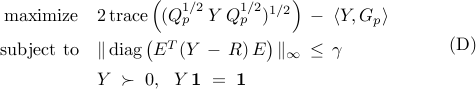where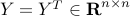is the dual variable associated with the equality constraint in (P).

### Growing connected resistive networks

The problem of topology identification and optimal design of stochastically-forced networks has many interesting variations. An important class of problems is given by resistive networks in which all edge weights are restricted to be non-negative. Here, we study the problem of growing connected resistive networks. In this, the plant graph is connected and there are no joint edges between the plant and the controller graphs. In the absence of the sparsity-promoting term, the closed-loop network is given by a complete graph. Our objective is to add a small number of edges in order to optimally enhance the closed-loop performance.

The restriction on connected plant graphs implies positive definiteness of the strengthened graph Laplacian of the plant,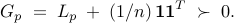Thus,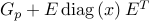is always positive definite for connected resistive networks. As done in problem (P), in order to determine the Lagrange dual of the optimization problem, we introduce an additional optimization variable. The sparsity-promoting optimal control problem simplifies to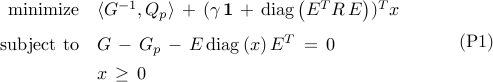where the matrixand the vectorare optimization variables. Non-negativity of the edge weights was used to write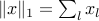and to remove positive definite requirements on.

The Lagrange dual of the sparsity-promoting optimal control problem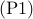is given by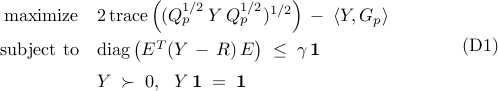where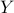is the dual variable associated with the equality constraint in.

## Acknowledgements

This project is supported by the